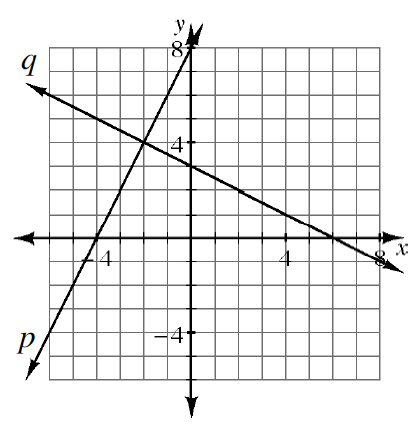### Home > GC > Chapter 2 > Lesson 2.3.3 > Problem2-119

2-119.

Lines $p$ and $q$ graphed in problem 2-118 form a triangle with the $x$-axis.

1. How can you describe this triangle? In other words, what is the most appropriate name for this triangle? How do you know?

What do you notice about the slopes of the lines?2. Find the area of the triangle.

$\text{Area = }\frac{1}{2}(\text{base})(\text{height})$

3. What is the perimeter?

The perimeter is $\approx23.4$ units.